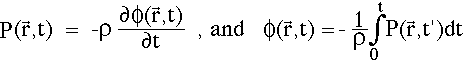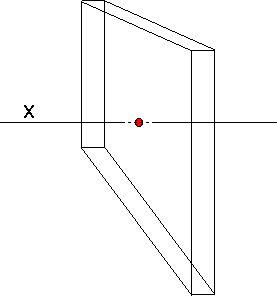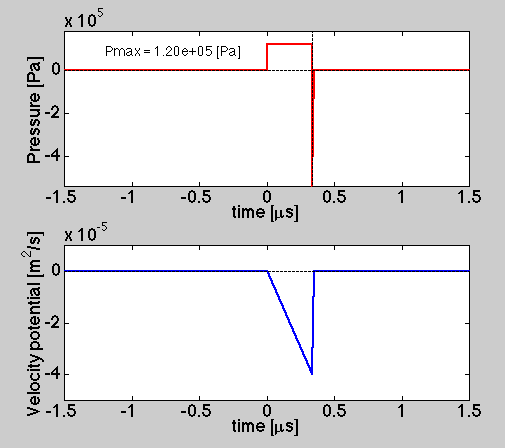# Pressure related to Velocity Potential

The pressure (P [Pa]) is related to the change with respect to time of a parameter called the velocity potential φ [m2/s]):where ρ is density [kg/m3], r is the position of observation, and t is time [s].

To illustrate P and φ, consider a position in the middle of a 1-mm-thick slab of absorbing gel within an infinite medium of water. The slab has an energy deposition = 1 [J/cm3] = 106 [J/m3] equivalent to a 0.24 degree C temperature jump. Assume the Grueneisen coefficient Γ is 0.12 and the density ρ is 1 [kg/m3], both typical for water at 25 degree C. The following figure plots the pressure and velocity potential as functions of time. The laser pulse occurs at t = 0.FIGURE: Consider the pressure and velocity potential in the center of a thin 1-mm slab (consider x-axis behavior). At time zero, the center of the slab is a distance of 0.5 mm from the front and rear surfaces. The initial pressure throughout the slab is 1.2x105 [Pa] or 1.2 bars. The pressure releases at the front and rear surfaces of the slab travel at the speed of sound (1.5 mm/us) and arrive at our observation point in the center at (0.5 mm)/(1.5 mm/μs) = 0.333 μs after the laser pulse. At that time the pressure goes very negative (off the scale of figure) as a negative impulse and the velocity potential is brought back to zero.